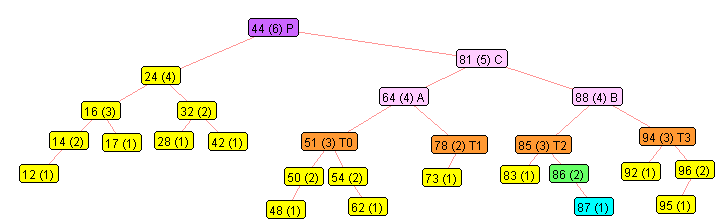# The AVL algorithm for balancing a Binary Search Tree.

## By Nicholas Duchon, Oct 14, 2011

Much of this presentation follows the presentation of AVL trees in Data Structures and Algorithms in Java, 3rd edition, pp 421-431, ISBN 0-471-46983-1, 2004.

Let us assume that the BST is defined with:
• left < node < right
We will not allow repeats in these trees.

The algorithm uses a balance factor at each node based on the height of that node. So we need to define these terms.
• Height:
• The height of a node is one more than the length of the longest path from the node to a leaf of the subtree with the node as the root.
• Thus, the height of a leaf is 1.
• The height of null children is 0.
• Balance Factor:
• The balance factor of a node in a binary tree is the absolute value of the height of the left child minus the height of the right child.
• AVL Balanced:
• A binary search tree is AVL balanced if the balance factor at every node is no more than 1, meaning either 0 or 1.
For example, I have labelled the following tree with values and the heights of the nodes in parentheses.
You can generate this tree adding nodes in the level order, the first is the original tree, and the second adds the unbalanced node 87.
I have used space delimiters since that is the default for Scanner, and using Scanner.nextInt becomes simple.
• 44 24 64 16 32 51 88 14 17 28 42 50 54 81 94 12 48 62 78 85 92 96 73 83 86 95
• 44 24 64 16 32 51 88 14 17 28 42 50 54 81 94 12 48 62 78 85 92 96 73 83 86 95 87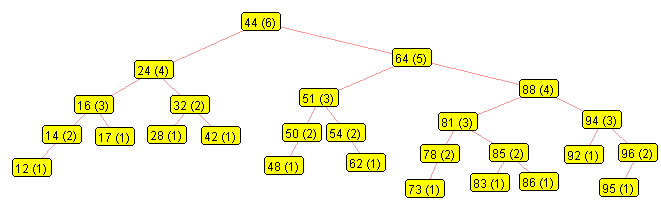All the nodes in the tree above are balanced.

We will now add 87 to the tree, and from that node up, we recompute the heights and balance factors.

We begin with the node just inserted and go up the tree looking for a node that is not balanced:
• 87 - children are (0, 0), so balanced, go to parent
• 86 - children are (0, 1), so balanced, go to parent
• 85 - children are (1, 2), so balanced, go to parent
• 81 - children are (2, 3), so balanced, go to parent
• 88 - children are (4, 3), so balanced, go to parent
• 64 - children are (3, 5), so NOT balanced,
• so start AVL balancing algorithm at node 64,
• keeping track of child and grandchild nodes in path: (64, 88, 81), and
• we need to keep track of the other children of those nodes, which we will call T0, T1, T2 and T3.
In this case, we see that node 64 is not balanced since the heights of its children are 3 and 5 respectively.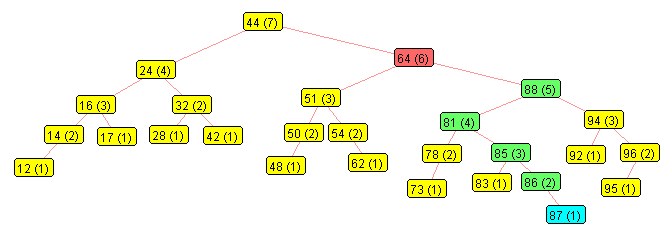Here, we have labelled those nodes:
• A (64) - the node where the unbalance was detected
• B (88) - that node's child, which we tracked during the upward path from the inserted node (87)
• C (81) - the unbalanced node's grandchild, also tracked during the upward path from (87)
• T0, T1, T2 and T3 - the other children of nodes A, B and C
• T0 (51) - the other child of A
• T1 (78) - one child of C
• T2 (85) - the other child of C
• T3 (94) - the other child of B
• P (44) - the parent of the unbalanced node.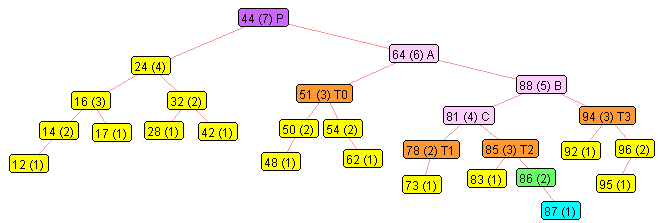Algorithm:
• Order A, B and C. In this case:
• A --> C --> B  (64 --> 81 --> 88)
• Order T0, T1, T2 and T3. In this case, the order is the same:
• T0 --> T1 --> T2 --> T3
• Reconnect the tree:
• A.parent.right = C, the middle of {A, B, C}
• C.left = A, smallest of {A, B, C}
• C.right = B, largest of {A, B, C}
• A.left = T0, smallest of {T0, T1, T2, T3}
• A.right = T1, next of {T0, T1, T2, T3}
• B.left = T2, next of {T0, T1, T2, T3}
• B.right = T3, largest of {T0, T1, T2, T3}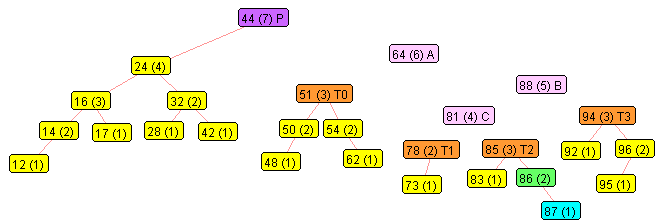### Ordering {A, B, C}: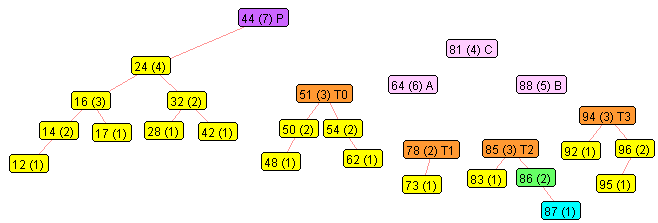### Connection P to middle, then middle to left and right of {A, B, C}: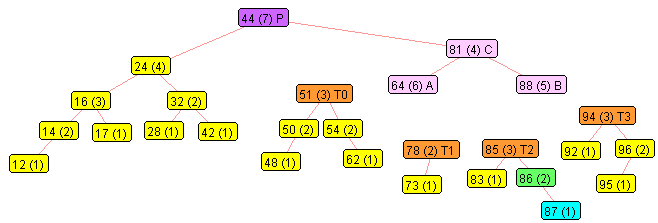### Moving the T's around a little: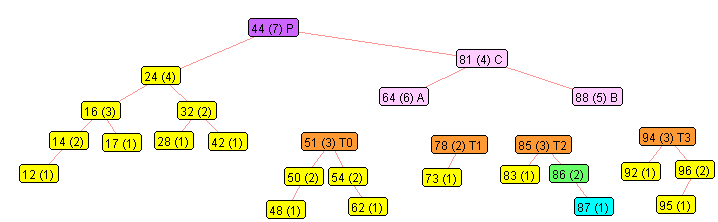### Connecting the T's: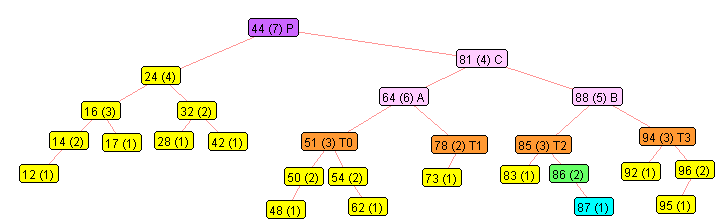### Recomputing the heights

• From the T's, so we recompute A, B (the children of the middle node), then C (the middle node), and then continue computing heights of parents of C (middle node). Now we see that everything is balanced.